# plot

## Syntax

``plot(t)``
``fig = plot(t)``
``newt = plot(trobj,'read',fig)``

## Description

`plot` is a graphical tree-management utility.

example

````plot(t)` plots the tree `t`. The figure that contains the tree is a GUI tool. You can change the Node Label to Depth_Position (default) or Index.You can change the Node Action to Visualize (default) or Split-Merge. You can click the nodes to execute the current Node Action.```
````fig = plot(t)` returns the handle to the figure.```
````newt = plot(trobj,'read',fig)` returns the tree plotted in `fig`. You can use this syntax to return the tree after performing some split or merge actions.```

## Examples

collapse all

This example shows how to plot wavelet packet trees.

`load noisbloc`

Obtain the `wptree` object that corresponds to a level 2 wavelet packet decomposition of the signal using the `db2` wavelet.

```x = noisbloc; t = wpdec(x,2,'db2');```

Plot the tree.

`plot(t)`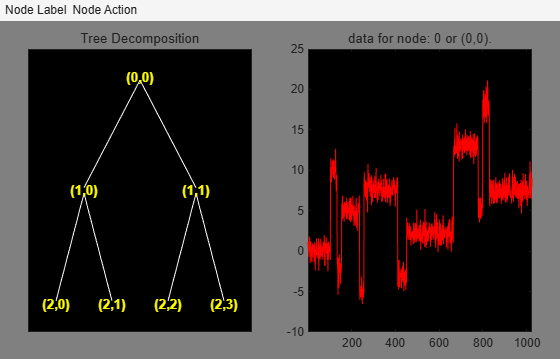Change Node Label from Depth_Position to Index. Click node (3) to obtain the following figure.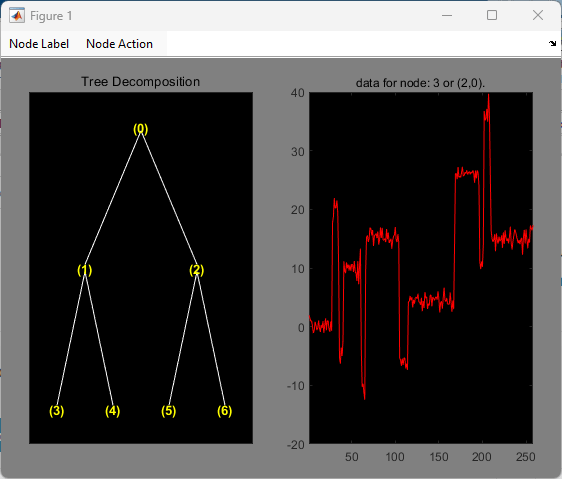Set the Node Label back to Depth_Position. Change Node Action to Split-Merge. Click on the `(1,1)` node to get the following figure. The figure shows the discrete wavelet transform down to level 2.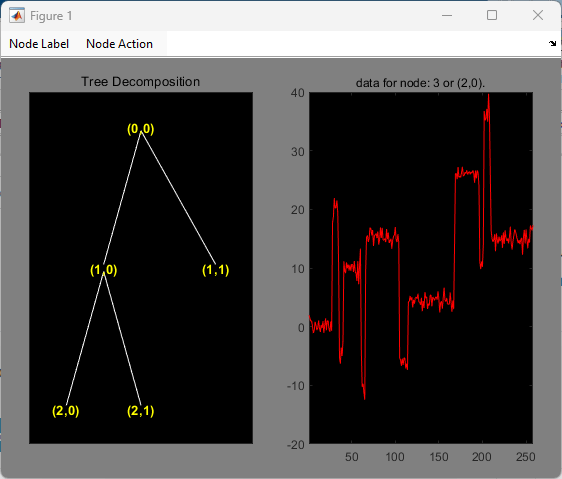Load an image. Obtain the `wptree` object that corresponds to a level 1 wavelet packet decomposition of the image using the `sym4` wavelet.

```load woman2 t = wpdec2(X,1,'sym4');```

Plot the tree.

`plot(t)`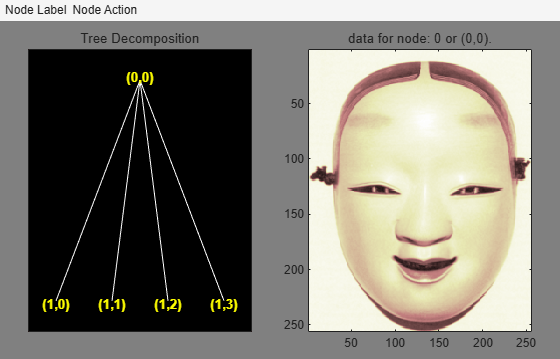Change Node Label from Depth_Position to Index. Click the node `(1)`. You get the following figure.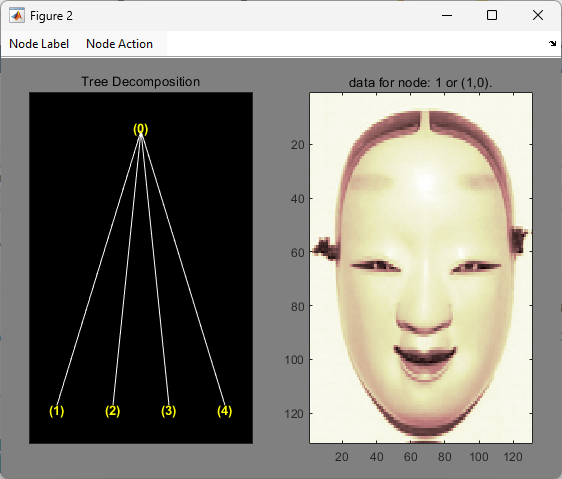## Input Arguments

collapse all

Tree, specified as an `ntree`, `dtree`, or `wptree` object.

Object, specified as an `NTREE`-parented object. `trobj` is an object constructor name returning an `NTREE`-parented object.

Example: `newt = plot(ntree,'read',fig)`, ```newt = plot(dtree,'read',fig)```, ```newt = plot(wptree,'read',fig)```

## Output Arguments

collapse all

Figure containing plot, returned as a `Figure` object.

## Version History

Introduced before R2006a